#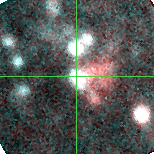V-120805 (J013411.58+303617.4)

## Target Notes### Classification: -

Not resolved clearly from J013411.48+303615.0 about 1 magnitude fainter 2.7 arcseconds SW. Also a blend of three separate stars combined to about 1 magnitude fainter 3 arcseconds to the south. Big red clump to the SW could be a partly obscured star cluster.

## Plots of Brightness and Color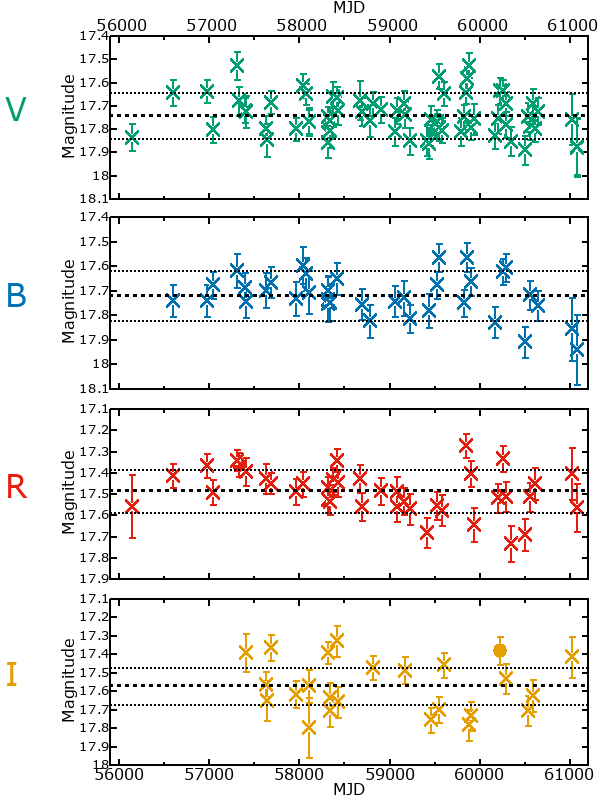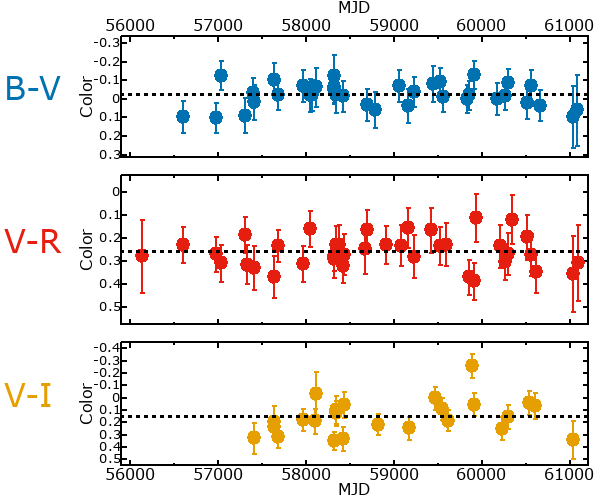## Variability

From the data available in this survey, it is not possible to tell with certainty if this star is variable.

### Correlation factors between bands

If the brightness variation is true variability then there should be a correlation between brightness changes recorded in different filter bands. The following table are the R-squared linear correlation factors calculated for the pairs of bands. The number in parentheses next to the R-squared value is the number of elements used to calculate the correlation factor. The number in square brackets next to the band is the range of magnitudes measured.

V [0.21 mag]B [0.35 mag]R [0.24 mag]
B [0.21 mag]0.23 (22)
R [0.24 mag]0.54 (23)0.43 (16)
I [0.46 mag]0.49 (14)0.31 (8)0.65 (8)

### Magnitude-Magnitude Plots

The plots below are provided to check the values in the table above and also to show any non-linear correlations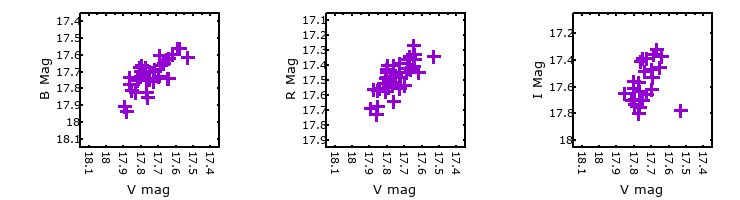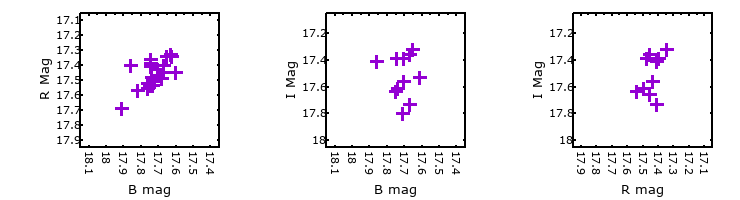### I & R Statistics

Welch & Stetson (1993, AJ, 105, 1813) present a method for varible star detection in CCD images from automated surveys. Data from two bands are compared and two statistics are calculated: R and I.

The I-statistic has an expectation value of zero for stars that are NOT variable. A larger non-zero I-value indicates a higher likelihood that the star is variable. The R statistic indicates how the color of the star changes as the variable grows brighter with R = 1.0 being no change in color.

R and I values have only been calculated if there are more than four (4) matched measurements of brightness in each band.
V
(I|R)
B
(I|R)
R
(I|R)
B
(I|R)
0.58 | 0.63
R
(I|R)
0.99 | 1.210.97 | 0.17
I
(I|R)
0.93 | 0.440.79 | 0.281.48 | 0.43